{{ message }}

# d3 / d3-delaunay

Compute the Voronoi diagram of a set of two-dimensional points.

Switch branches/tags
Nothing to show

## Files

Failed to load latest commit information.
Type
Name
Commit time
Sep 12, 2020
Mar 21, 2018
Apr 20, 2021
Aug 24, 2018
Mar 19, 2018
Jan 11, 2018
Aug 14, 2020
Aug 24, 2018
May 28, 2020
Jun 19, 2019
Aug 30, 2019

# d3-delaunay

Georgy “The Voronator” Voronoy

This is a fast library for computing the Voronoi diagram of a set of two-dimensional points. It is based on Delaunator, a fast library for computing the Delaunay triangulation using sweep algorithms. The Voronoi diagram is constructed by connecting the circumcenters of adjacent triangles in the Delaunay triangulation.

For an interactive explanation of how this library works, see The Delaunay’s Dual.

## Installing

To install, `npm install d3-delaunay` or `yarn add d3-delaunay`. You can also download the latest release or load directly from unpkg. AMD, CommonJS and ES6+ environments are supported. In vanilla, a `d3` global is exported.

```import {Delaunay} from "d3-delaunay";

const points = [[0, 0], [0, 1], [1, 0], [1, 1]];
const delaunay = Delaunay.from(points);
const voronoi = delaunay.voronoi([0, 0, 960, 500]);```

## API Reference

### Delaunay

# new Delaunay(points) <>

Returns the Delaunay triangulation for the given flat array [x0, y0, x1, y1, …] of points.

`const delaunay = new Delaunay(Float64Array.of(0, 0, 0, 1, 1, 0, 1, 1));`

# Delaunay.from(points[, fx[, fy[, that]]]) <>

Returns the Delaunay triangulation for the given array or iterable of points. If fx and fy are not specified, then points is assumed to be an array of two-element arrays of numbers: [[x0, y0], [x1, y1], …]. Otherwise, fx and fy are functions that are invoked for each element in the points array in order, and must return the respective x- and y-coordinate for each point. If that is specified, the functions fx and fy are invoked with that as this. (See Array.from for reference.)

`const delaunay = Delaunay.from([[0, 0], [0, 1], [1, 0], [1, 1]]);`

# delaunay.points

The coordinates of the points as an array [x0, y0, x1, y1, …]. Typically, this is a Float64Array, however you can use any array-like type in the constructor.

# delaunay.halfedges

The halfedge indexes as an Int32Array [j0, j1, …]. For each index 0 ≤ i < halfedges.length, there is a halfedge from triangle vertex j = halfedges[i] to triangle vertex i. Equivalently, this means that triangle ⌊i / 3⌋ is adjacent to triangle ⌊j / 3⌋. If j is negative, then triangle ⌊i / 3⌋ is an exterior triangle on the convex hull. For example, to render the internal edges of the Delaunay triangulation:

```const {points, halfedges, triangles} = delaunay;
for (let i = 0, n = halfedges.length; i < n; ++i) {
const j = halfedges[i];
if (j < i) continue;
const ti = triangles[i];
const tj = triangles[j];
context.moveTo(points[ti * 2], points[ti * 2 + 1]);
context.lineTo(points[tj * 2], points[tj * 2 + 1]);
}```

# delaunay.hull

An Int32Array of point indexes that form the convex hull in counterclockwise order. If the points are collinear, returns them ordered.

# delaunay.triangles

The triangle vertex indexes as an Uint32Array [i0, j0, k0, i1, j1, k1, …]. Each contiguous triplet of indexes i, j, k forms a counterclockwise triangle. The coordinates of the triangle’s points can be found by going through delaunay.points. For example, to render triangle i:

```const {points, triangles} = delaunay;
const t0 = triangles[i * 3 + 0];
const t1 = triangles[i * 3 + 1];
const t2 = triangles[i * 3 + 2];
context.moveTo(points[t0 * 2], points[t0 * 2 + 1]);
context.lineTo(points[t1 * 2], points[t1 * 2 + 1]);
context.lineTo(points[t2 * 2], points[t2 * 2 + 1]);
context.closePath();```

# delaunay.inedges

The incoming halfedge indexes as a Int32Array [e0, e1, e2, …]. For each point i, inedges[i] is the halfedge index e of an incoming halfedge. For coincident points, the halfedge index is -1; for points on the convex hull, the incoming halfedge is on the convex hull; for other points, the choice of incoming halfedge is arbitrary. The inedges table can be used to traverse the Delaunay triangulation; see also delaunay.neighbors.

# delaunay.find(x, y[, i]) <>

Returns the index of the input point that is closest to the specified point ⟨x, y⟩. The search is started at the specified point i. If i is not specified, it defaults to zero.

# delaunay.neighbors(i) <>

Returns an iterable over the indexes of the neighboring points to the specified point i. The iterable is empty if i is a coincident point.

# delaunay.render([context]) <>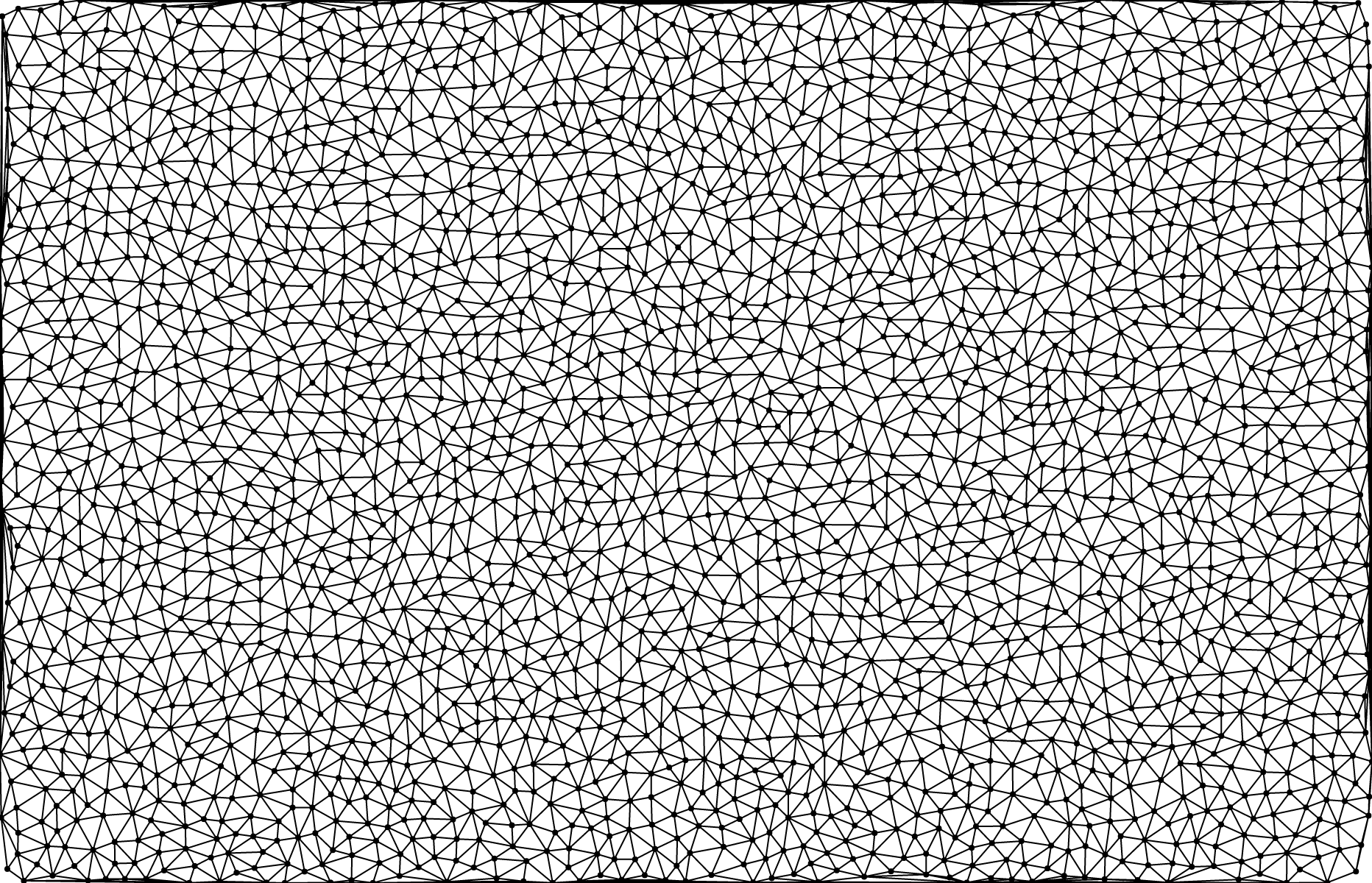Renders the edges of the Delaunay triangulation to the specified context. The specified context must implement the context.moveTo and context.lineTo methods from the CanvasPathMethods API. If a context is not specified, an SVG path string is returned instead.

# delaunay.renderHull([context]) <>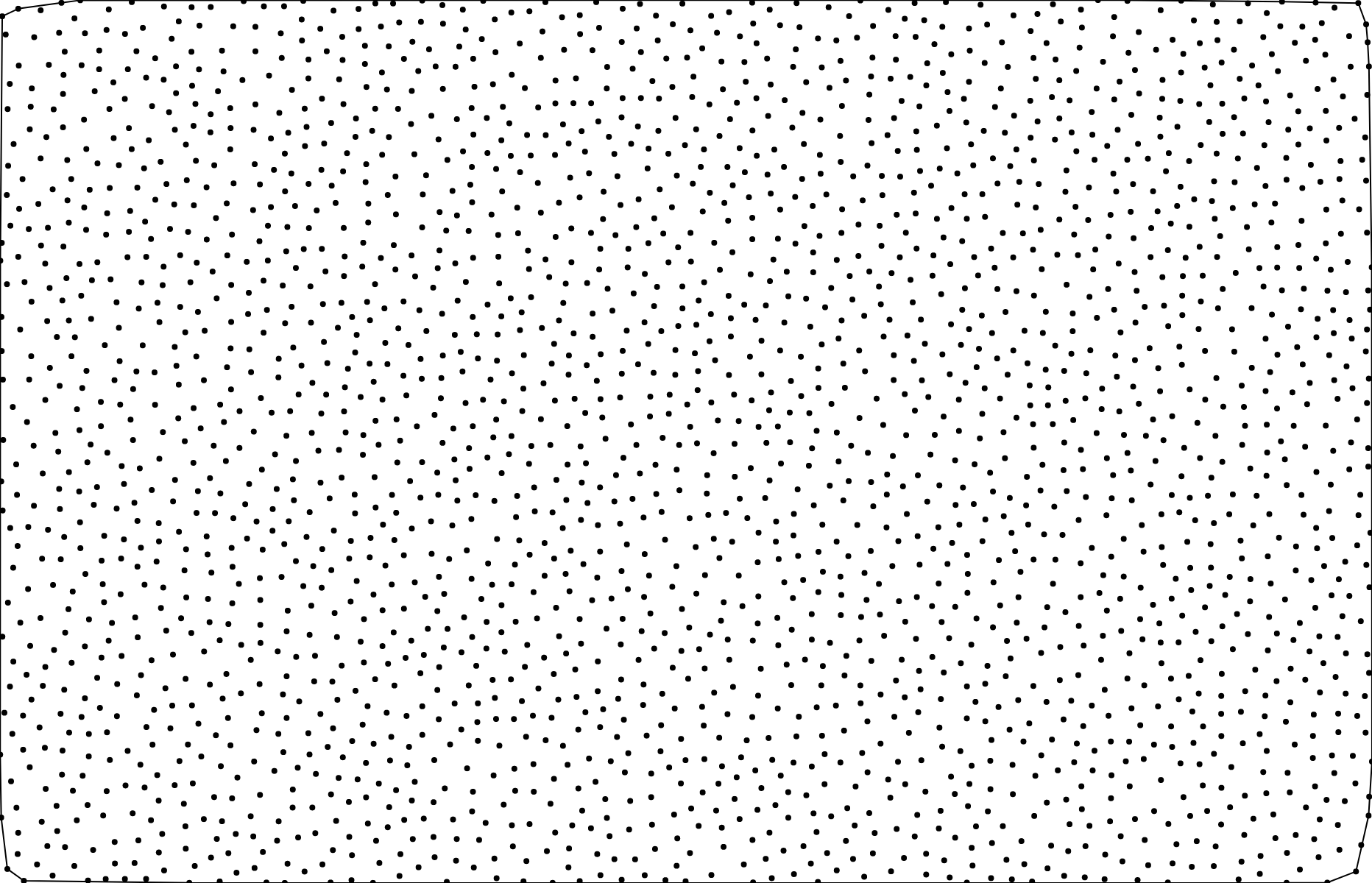Renders the convex hull of the Delaunay triangulation to the specified context. The specified context must implement the context.moveTo and context.lineTo methods from the CanvasPathMethods API. If a context is not specified, an SVG path string is returned instead.

# delaunay.renderTriangle(i[, context]) <>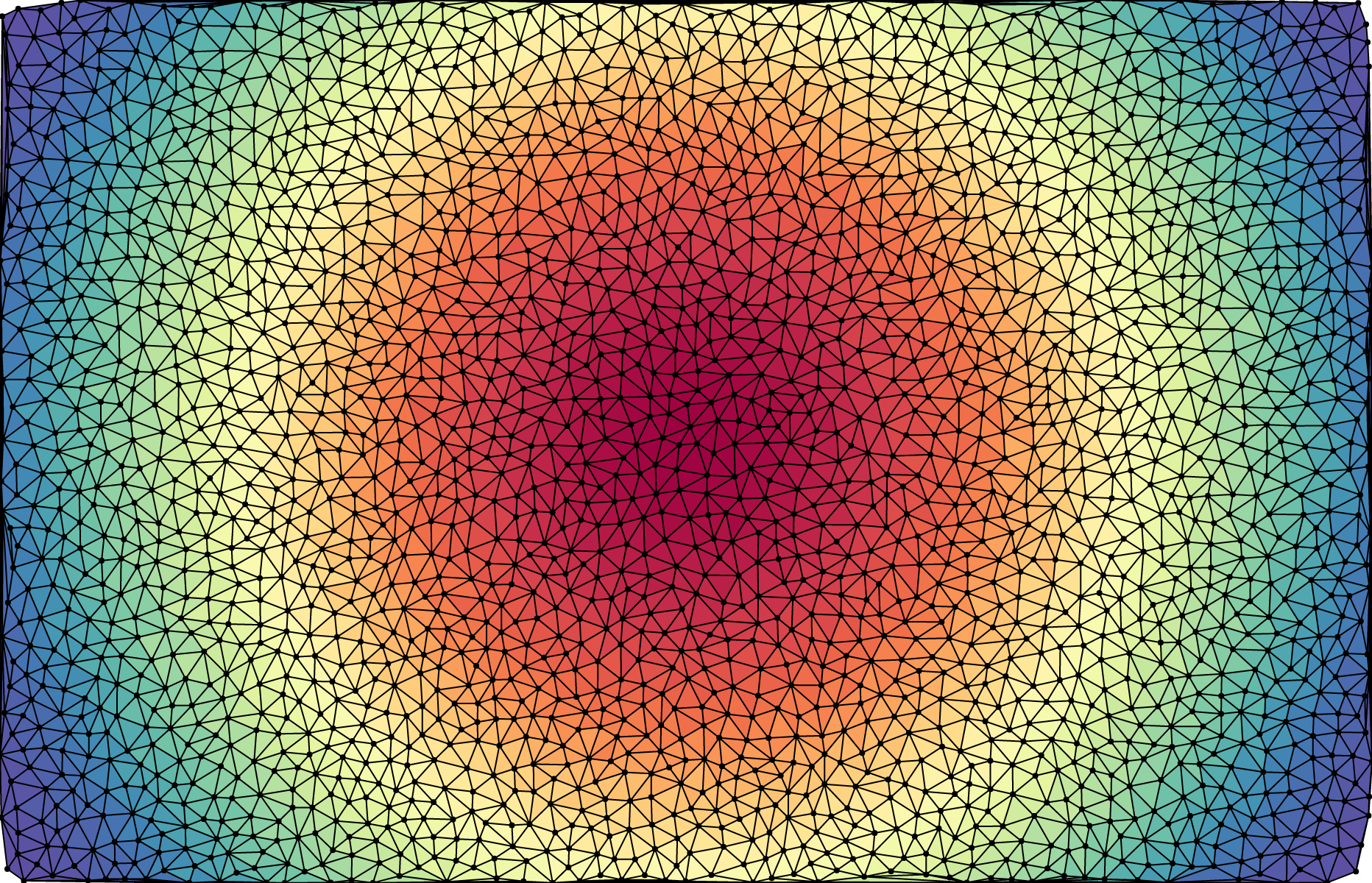Renders triangle i of the Delaunay triangulation to the specified context. The specified context must implement the context.moveTo, context.lineTo and context.closePath methods from the CanvasPathMethods API. If a context is not specified, an SVG path string is returned instead.

Renders the input points of the Delaunay triangulation to the specified context as circles with the specified radius. If radius is not specified, it defaults to 2. The specified context must implement the context.moveTo and context.arc methods from the CanvasPathMethods API. If a context is not specified, an SVG path string is returned instead.

# delaunay.hullPolygon() <>

Returns the closed polygon [[x0, y0], [x1, y1], …, [x0, y0]] representing the convex hull.

# delaunay.trianglePolygons() <>

Returns an iterable over the polygons for each triangle, in order.

# delaunay.trianglePolygon(i) <>

Returns the closed polygon [[x0, y0], [x1, y1], [x2, y2], [x0, y0]] representing the triangle i.

# delaunay.update() <>

Updates the triangulation after the points have been modified in-place.

# delaunay.voronoi([bounds]) <>

Returns the Voronoi diagram for the associated points. When rendering, the diagram will be clipped to the specified bounds = [xmin, ymin, xmax, ymax]. If bounds is not specified, it defaults to [0, 0, 960, 500]. See To Infinity and Back Again for an interactive explanation of Voronoi cell clipping.

The Voronoi diagram is returned even in degenerate cases where no triangulation exists — namely 0, 1 or 2 points, and collinear points.

### Voronoi

# voronoi.delaunay

The Voronoi diagram’s associated Delaunay triangulation.

# voronoi.circumcenters

The circumcenters of the Delaunay triangles as a Float64Array [cx0, cy0, cx1, cy1, …]. Each contiguous pair of coordinates cx, cy is the circumcenter for the corresponding triangle. These circumcenters form the coordinates of the Voronoi cell polygons.

# voronoi.vectors

A Float64Array [vx0, vy0, wx0, wy0, …] where each non-zero quadruple describes an open (infinite) cell on the outer hull, giving the directions of two open half-lines.

# voronoi.xmin
# voronoi.ymin
# voronoi.xmax
# voronoi.ymax

The bounds of the viewport [xmin, ymin, xmax, ymax] for rendering the Voronoi diagram. These values only affect the rendering methods (voronoi.render, voronoi.renderBounds, cell.render).

# voronoi.contains(i, x, y) <>

Returns true if the cell with the specified index i contains the specified point ⟨x, y⟩. (This method is not affected by the associated Voronoi diagram’s viewport bounds.)

# voronoi.neighbors(i) <>

Returns an iterable over the indexes of the cells that share a common edge with the specified cell i. Voronoi neighbors are always neighbors on the Delaunay graph, but the converse is false when the common edge has been clipped out by the Voronoi diagram’s viewport.

# voronoi.render([context]) <>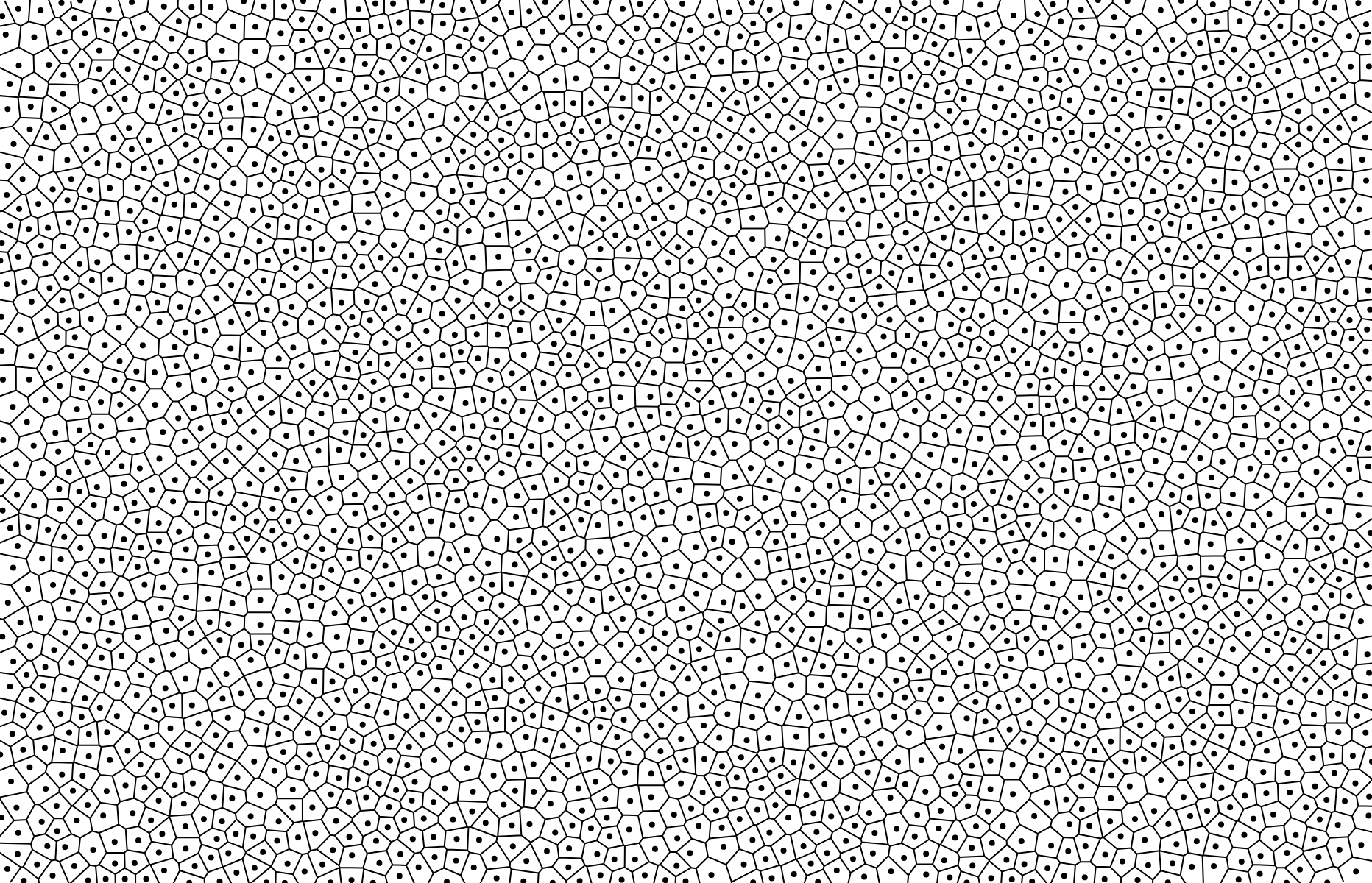Renders the mesh of Voronoi cells to the specified context. The specified context must implement the context.moveTo and context.lineTo methods from the CanvasPathMethods API. If a context is not specified, an SVG path string is returned instead.

# voronoi.renderBounds([context]) <>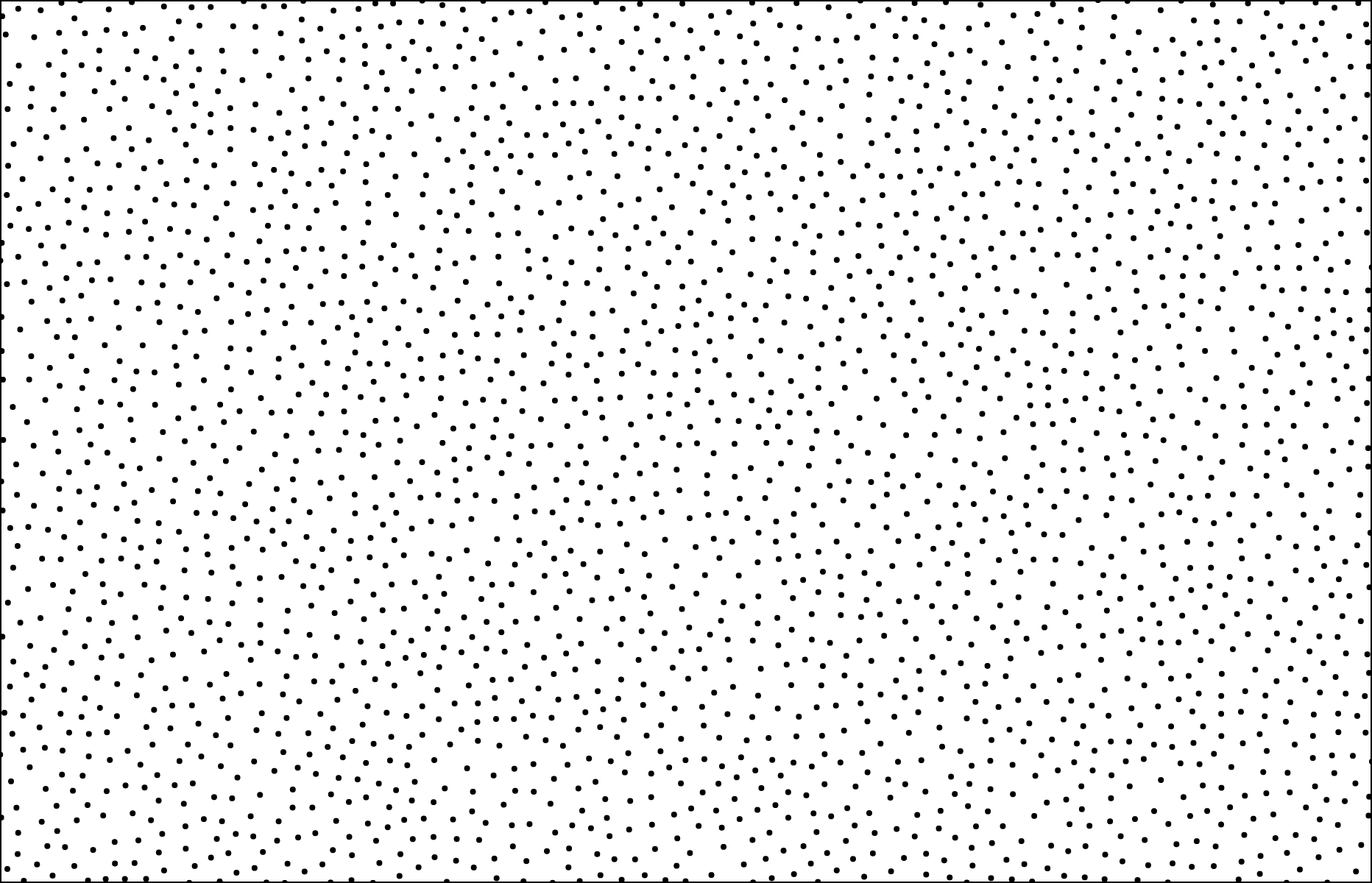Renders the viewport extent to the specified context. The specified context must implement the context.rect method from the CanvasPathMethods API. Equivalent to context.rect(voronoi.xmin, voronoi.ymin, voronoi.xmax - voronoi.xmin, voronoi.ymax - voronoi.ymin). If a context is not specified, an SVG path string is returned instead.

# voronoi.renderCell(i[, context]) <>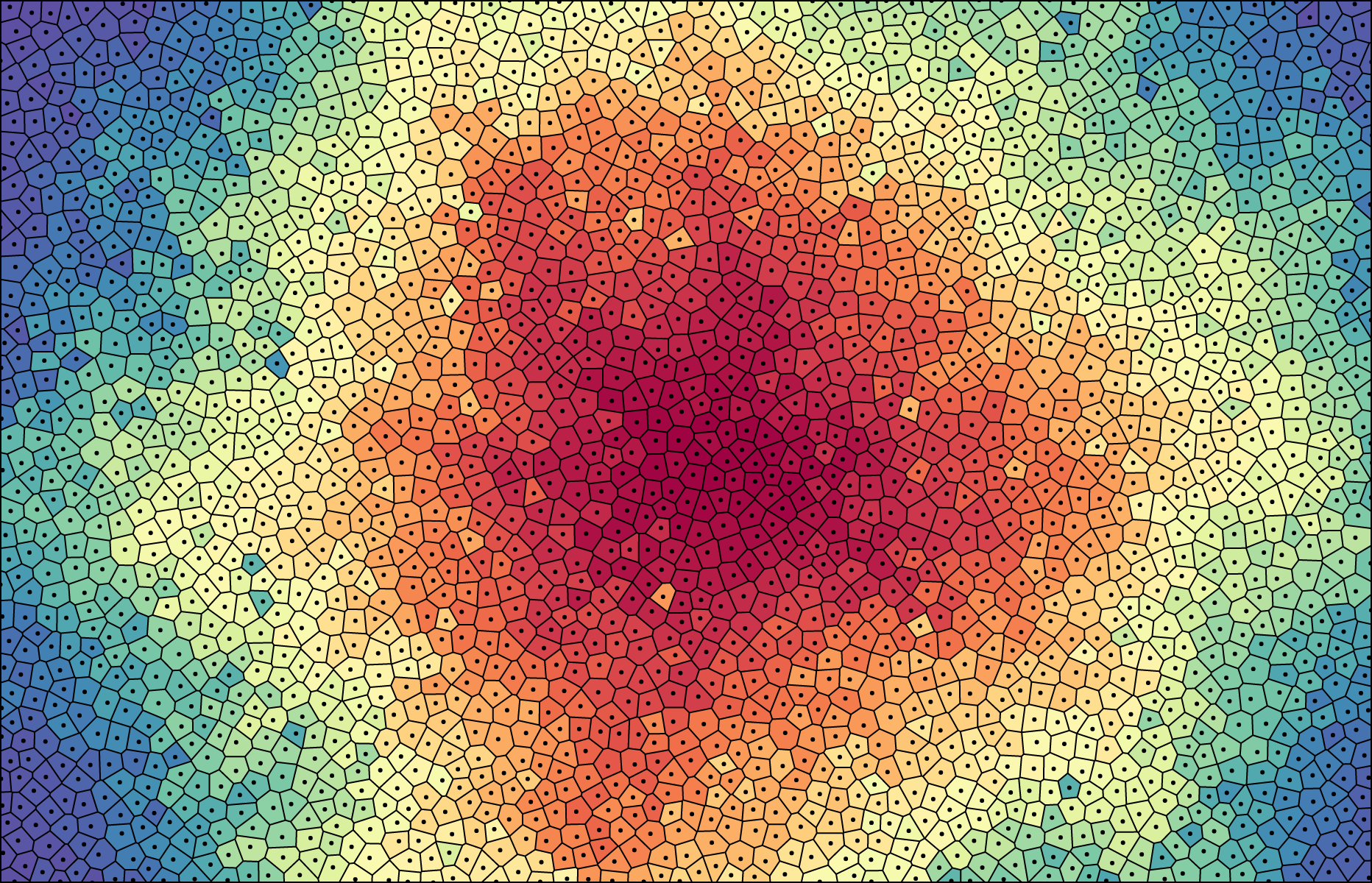Renders the cell with the specified index i to the specified context. The specified context must implement the context.moveTo , context.lineTo and context.closePath methods from the CanvasPathMethods API. If a context is not specified, an SVG path string is returned instead.

# voronoi.cellPolygons() <>

Returns an iterable over the non-empty polygons for each cell, with the cell index as property.

# voronoi.cellPolygon(i) <>

Returns the convex, closed polygon [[x0, y0], [x1, y1], …, [x0, y0]] representing the cell for the specified point i.

# voronoi.update() <>

Updates the Voronoi diagram and underlying triangulation after the points have been modified in-place — useful for Lloyd’s relaxation.

Compute the Voronoi diagram of a set of two-dimensional points.